Open in App
Not now

# Wait For Graph Deadlock Detection in Distributed System

• Last Updated : 28 Apr, 2022

Deadlocks are a fundamental problem in distributed systems. A process may request resources in any order and a process can request resources while holding others. A Deadlock is a situation where a set of processes are blocked as each process in a Distributed system is holding some resources and that acquired resources are needed by some other processes.

Example:

If there are three processes p1,p2 and p1 are acquiring r1 resource and that r1 is needed by p2 which is acquiring another resource r2 and that is needed by p1. Here cycle occurs. It is called a deadlock.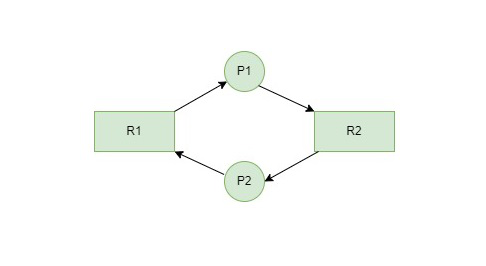Here in the above graph we found a cycle from P1 to P2 and again to P1. So we can say that the system is in a deadlock state.

The Problem of deadlocks has been generally studied in distributed systems under the following models :

• The system has only reusable resources.
• There is only one copy of each resource.

The deadlock Detection Algorithm is of two types:

• Wait-for-Graph Algorithm (Single Instance)
• Banker’s Algorithm (Multiple Instance)

Wait-for-Graph Algorithm: It is a variant of the Resource Allocation graph. In this algorithm, we only have processes as vertices in the graph. If the Wait-for-Graph contains a cycle then we can say the system is in a Deadlock state. Now we will discuss how the Resource Allocation graph will be converted into Wait-for-Graph in an Algorithmic Approach. We need to remove resources while converting from Resource Allocation Graph to Wait-for-Graph.

• Resource Allocation Graph: Contains Processes and Resources.
• Wait-for-Graph: Contains only Processes after removing the Resources while conversion from Resource Allocation Graph.

### Algorithm:

Step 1: Take the first process (Pi) from the resource allocation graph and check the path in which it is acquiring resource (Ri), and start a wait-for-graph with that particular process.

Step 2: Make a path for the Wait-for-Graph in which there will be no Resource included from the current process (Pi) to next process (Pj), from that next process (Pj) find a resource (Rj) that will be acquired by next Process (Pk) which is released from Process (Pj).

Step 3: Repeat Step 2 for all the processes.

Step 4: After completion of all processes, if we find a closed-loop cycle then the system is in a deadlock state, and deadlock is detected.

Now we will see the working of this Algorithm with an Example. Consider a Resource Allocation Graph with 4 Processes P1, P2, P3, P4, and 4 Resources R1, R2, R3, R4. Find if there is a deadlock in the Graph using the Wait for Graph-based deadlock detection algorithm.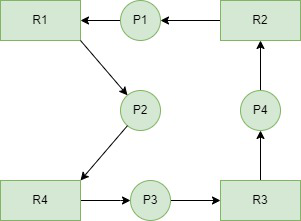Step 1: First take Process P1 which is waiting for Resource R1, resource R1 is acquired by Process P2, Start a Wait-for-Graph for the above Resource Allocation Graph.Step 2: Now we can observe that there is a path from P1 to P2 as P1 is waiting for R1 which is been acquired by P2. Now the Graph would be after removing resource R1 looks like.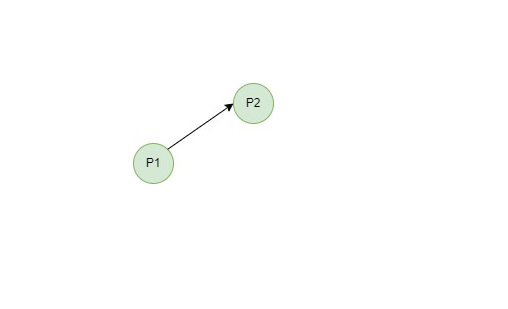Step 3: From P2 we can observe a path from P2 to P3 as P2 is waiting for R4 which is acquired by P3. So make a path from P2 to P3 after removing resource R4 looks like.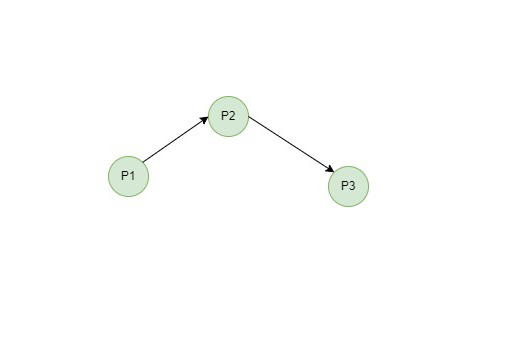Step 4: From P3 we find a path to P4 as it is waiting for P3 which is acquired by P4. After removing R3 the graph looks like this.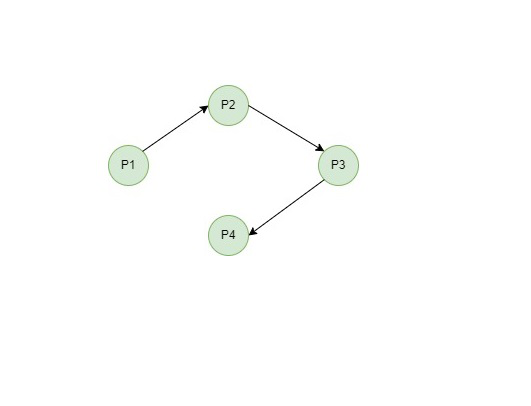Step 5: Here we can find Process P4 is waiting for R2 which is acquired by P1. So finally the Wait-for-Graph is as follows: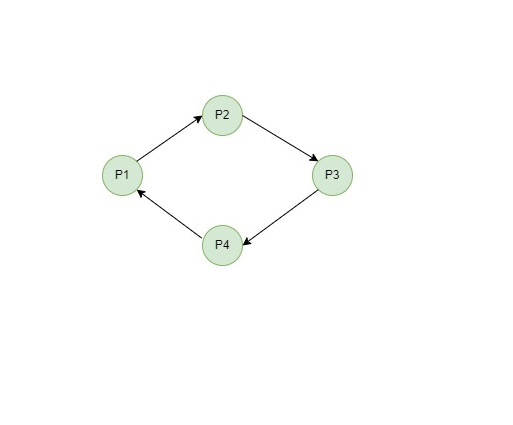Step 6: Finally In this Graph, we found a cycle as the Process P4 again came back to the Process P1 which is the starting point (i.e., it’s a closed-loop). So, According to the Algorithm if we found a closed loop, then the system is in a deadlock state. So here we can say the system is in a deadlock state.

Now consider another Resource Allocation Graph with 4 Processes P1, P2, P3, P4, and 3 Resources R1, R2, R3. Find if there is a deadlock in the Graph using the Wait for Graph-based deadlock detection algorithm.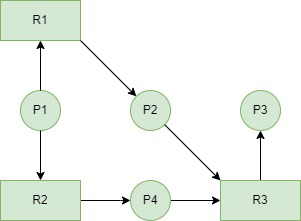Step 1: First take Process P1 which is waiting for Resource R1, resource R1 is acquired by Process P2, Start a Wait-for-Graph for the above Resource Allocation Graph.Step 2: Now we can observe that there is a path from P1 to P2 and also from P1 to P4 as P1 is waiting for R1 which is been acquired by P2 and P1 is also waiting for R2 which is acquired by P4. Now the Graph would be after removing resources R1 and R2 looks like.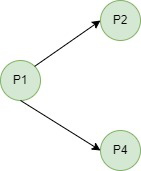Step 3: From P2 we can observe a path from P2 to P3 as P2 is waiting for R3 which is acquired by P3. So make a path from P2 to P3 after removing resource R3 looks like.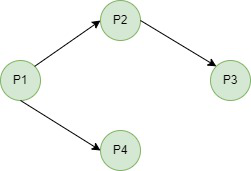Step 4: Here we can find Process P4 is waiting for R3 which is acquired by P3. So finally the Wait-for-Graph looks like after removing Resource R3 looks like.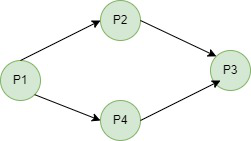Step 5: In this Graph, we don’t find a cycle as no process came back to the starting point (i.e., there is no closed loop). So, According to the Algorithm if we found a closed loop, then the system is in a deadlock state. But here we didn’t find any closed loop so the system is not in a deadlock state. The system is in a safe state.

Note: In Example 2, even though it looks like a loop but there is no process that has reached the first process, or starting point again. So there is no closed loop.

### Issues in Wait-for-Graph based Deadlock Detection Algorithm :

For every resource we call WFG Detection Algorithm, the computation time would be very high for the CPU if there are many resources. The solution for this is, rather than calling this algorithm for every resource request that cannot be granted immediately. Just invoke the algorithm after a definite interval.

My Personal Notes arrow_drop_up
Related Articles